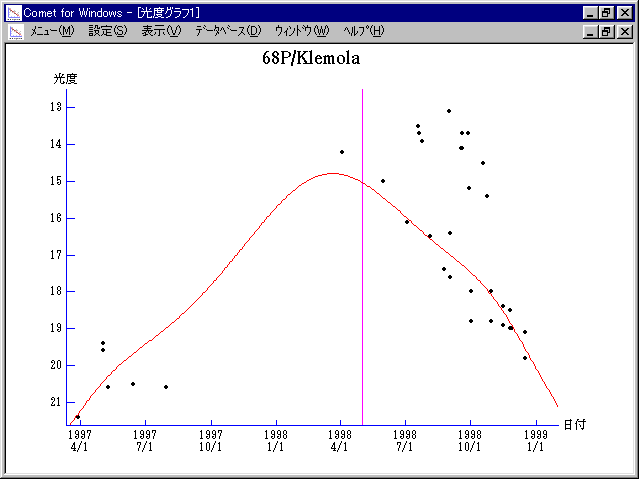# \$B%/%l%b%iWB@1(B

68P/Klemola (1998)###\$B50F;MWAG(B

```   The following improved orbital elements by Kenji Muraoka,
are from 161 observations  1965 to 1998, perturbations by
9 Plantes, Moon and 5 minor planets were taken into account.
The mean residual is +/- 0.99 arc seconds.

Epoch  =  1998 Apr. 17.0  TT       JDT = 2450920.5
T  =  1998 May   1.66765       +/- 0.00014 (m.e.) TT
Peri. =  154.54357                +/- 0.00008
Node  =  175.54315                +/- 0.00008   (2000.0)
Incl. =   11.08868                +/- 0.00004
q  =    1.7545181              +/- 0.0000007 AU
e  =    0.6413176              +/- 0.0000001
a  =    4.8915651              +/- 0.0000006 AU
n  =    0.09110293             +/- 0.00000002
P  =   10.819                  +/- 0.0000019  years
```

###\$B@1?^(B###\$B8wEYJQ2=(B

```        m1 = 7.7 + 5 log\$B&\$(B + 20.5 log r(t + 60)
```##### \$B50F;MWAG\$OB<2,7r<#;a\$N7W;;\$K\$h\$k\$b\$N\$G\$9!#(B \$B@1?^\$O(B StellaNavigator Ver.2.0 for Windows (\$B%"%9%H%m%"!<%D(B \$BJTCx(B / \$B%"%9%-!<=PHG6I4)(B) \$B\$G:n@.\$7\$?\$b\$N\$G\$9!#(B \$B8wEY%0%i%U\$O(BComet for Windows\$B\$G:n@.\$7\$?\$b\$N\$G\$9!#(B